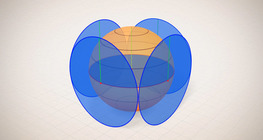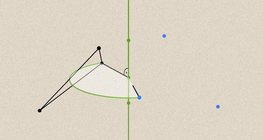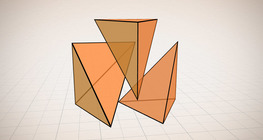Quantity: 0

Total: 0,00

0

# Volume of spheres (Cavalieri´s principle)

### Volume of spheres (Cavalieri´s principle)

Calculating the volume of a sphere is possible using an appropriate cylinder and cone.

Mathematics

Keywords

volume of sphere, Cavalieri´s principle, calculating volume, solids, sphere, mathematics

Related items

### Related items#### Sphere

A sphere is the set of points which are all within the same distance from a given point in space.#### Volume of spheres (demonstration)

The sum of the volume of the ´tetrahedrons´ gives an approximation of the volume of the sphere.#### Perimeter, area, surface area and volume

This animation presents the formulas to calculate the perimeter and area of shapes as well as the surface area and volume of solids.#### Physicists who changed the world

These great scientists had a tremendous impact on the advancement in physics.#### Ratio of volumes of similar solids

This 3D scene explains the correlation between the ratio of similarity and the ratio of volume of geometric solids.#### Surface area of spheres (demonstration)

The surface of a sphere consists of the set of points which are all at the same distance from a given point in space.#### Geometric transformations – rotation

This animation demonstrates geometric rotation, a type of geometric transformation both in plane and space.#### Solids of revolution

Rotating a geometric shape around a line within its geometric plane as an axis results in a solid of revolution.#### Volume and surface area (exercise)

An exercise about the volume and surface area of solids generated from a ´base cube´.#### Volume of a tetrahedron

To calculate the volume of a tetrahedron we start by calculating the volume of a prism.